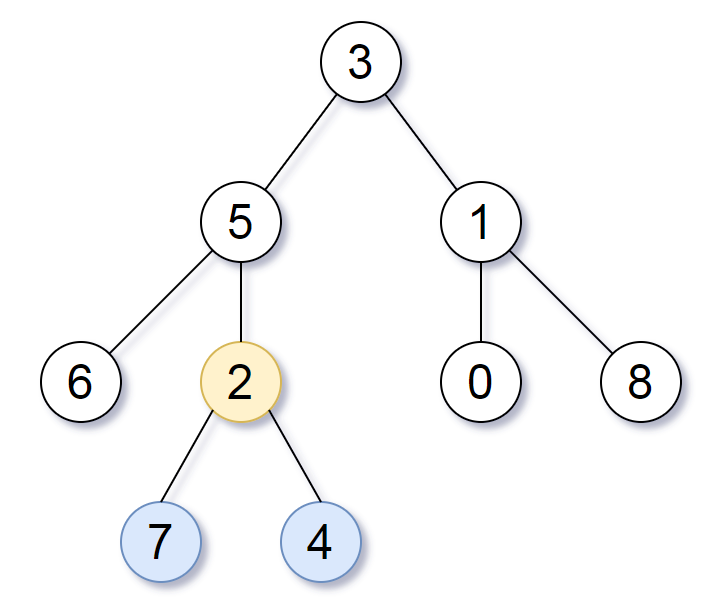Life of xhu

LeetCode 865 解析以及一个 JavaScript 作用域问题

Jul 20, 2018

|   #LeetCode   |   #JavaScript1. 如果左子节点为空, 那么左子树最大深度为当前节点深度, 不为空就遍历左子节点;
2. 如果右子节点为空, 那么右子树最大深度为当前节点深度, 不为空就遍历右子节点;
3. 如果两个子树最大深度相等, 那么返回当前节点, 否则就返回深度更大的子树.

``````/**
* Problem: https://leetcode.com/problems/smalle  st-subtree-with-all-the-deepest-nodes/description/
*/

const smallestSubtreeWithAllTheDeepestNodes = root => {
const traverse = (node, depth) => {
let subtreeL, subtreeR;
let depthL = depth;
let depthR = depth;

if (node.left) [subtreeL, depthL] = traverse(node.left, depth + 1);
if (node.right) [subtreeR, depthR] = traverse(node.right, depth + 1);

if (depthL === depthR)
return [node, depthL];
else
return depthL > depthR ? [subtreeL, depthL] : [subtreeR, depthR];
};

return traverse(root, 0);
};
``````

``````const smallestSubtreeWithAllTheDeepestNodes = root => {
const traverse = (node, depth) => {
let subtreeL, subtreeR;
let depthL = depthR = depth;

if (node.left) [subtreeL, depthL] = traverse(node.left, depth + 1);
if (node.right) [subtreeR, depthR] = traverse(node.right, depth + 1);

if (depthL === depthR)
return [node, depthL];
else
return depthL > depthR ? [subtreeL, depthL] : [subtreeR, depthR];
};

return traverse(root, 0);
};
``````

``````let depthL = depthR = depth;
``````

``````depthR = depth;
let depthL = depthR;
``````